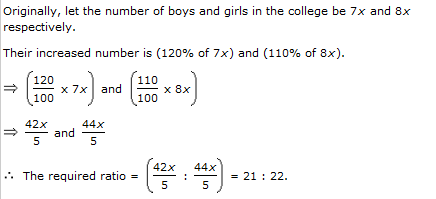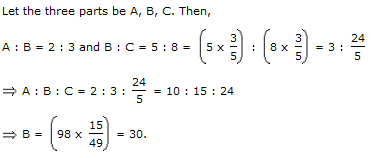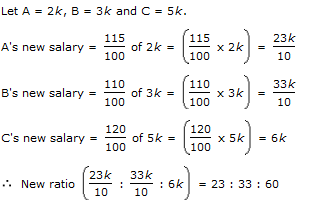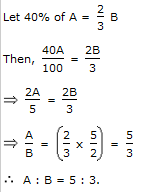6.

The ratio of the number of boys and girls in a college is 7 : 8. If the percentage increase in the number of boys and girls be 20% and 10% respectively, what will be the new ratio?

 A. 8 : 9 B. 17 : 18 C. 21 : 22 D. Cannot be determined
Explanation:7.

If 0.75 : x :: 5 : 8, then x is equal to:

 A. 1.12 B. 1.2 C. 1.25 D. 1.3
Explanation: (x x 5) = (0.75 x 8) x = 6/5 = 1.20

8.

The sum of three numbers is 98. If the ratio of the first to second is 2 :3 and that of the second to the third is 5 : 8, then the second number is:

 A. 20 B. 30 C. 48 D. 58
Explanation:9.

The salaries A, B, C are in the ratio 2 : 3 : 5. If the increments of 15%, 10% and 20% are allowed respectively in their salaries, then what will be new ratio of their salaries?

 A. 3 : 3 : 10 B. 10 : 11 : 20 C. 23 : 33 : 60 D. Cannot be determinedExplanation: